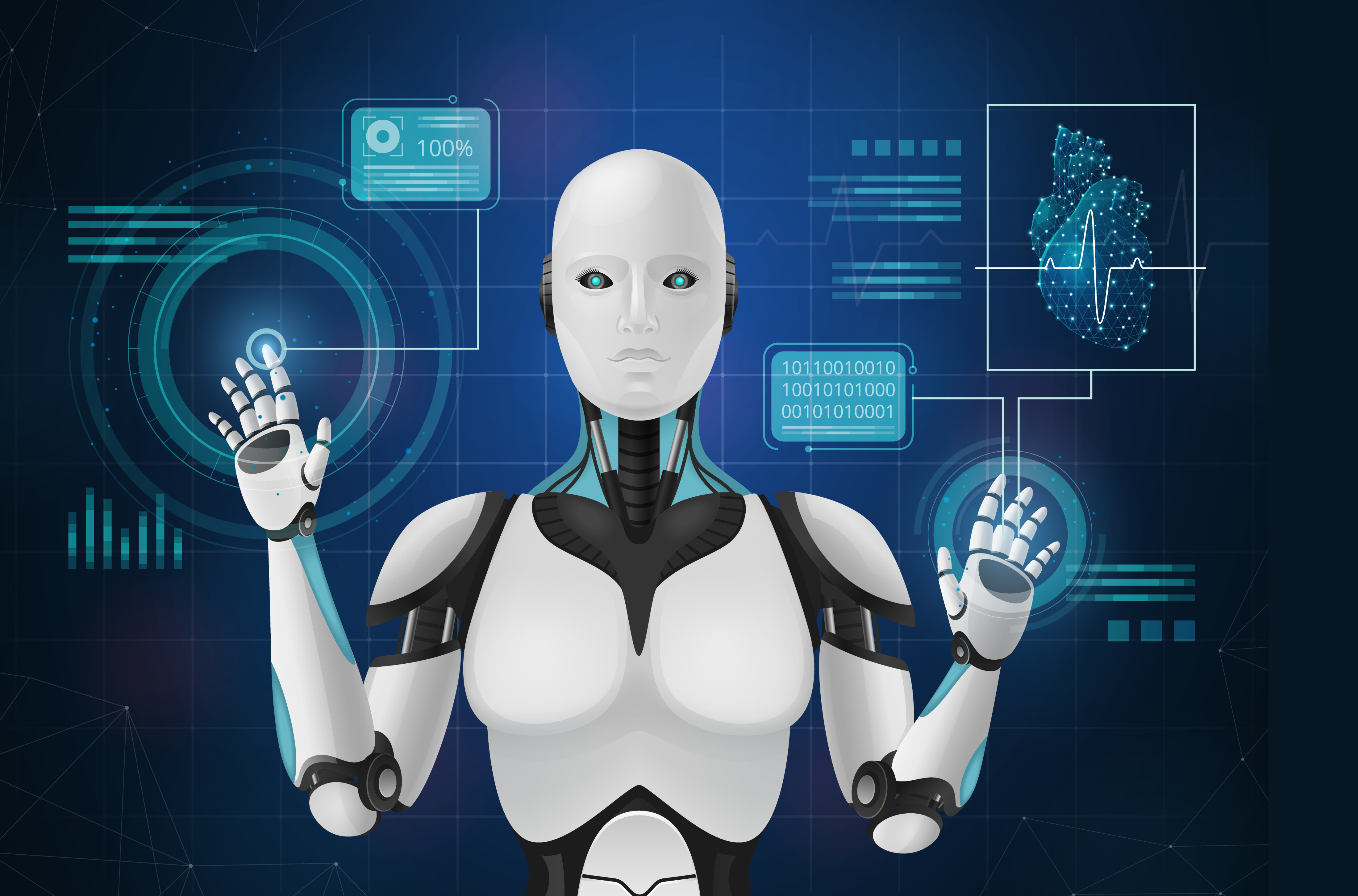• by Team Handson
• July 26, 2022

## Introduction To Machine Learning with Python

What is Machine Learning?

“The field of study that gives computers the ability to learn without being explicitly programmed”– Arthur Samuel

“A computer program is said to learn from experience ‘E’ with respect to some class of tasks ‘T’ and performance measure ‘P’, if its performance at tasks in T, as measured by P, improves with experience E.”– Tom M. Mitchell

Let’s try to understand the definition given by Tom Mitchell with couple of examples…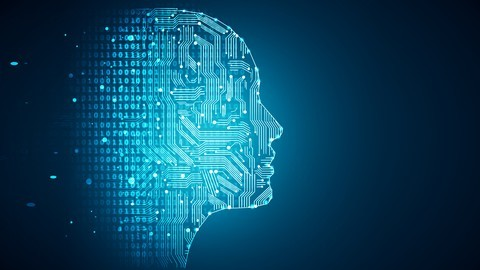The Complete Machine Learning Course with Python

Example-1:

Classifying Emails as Spam or Not Spam:

• Task (T): Classifying emails as spam or not spam.
• Experience (E): Watching the user to mark/label the emails as spam or not spam.
• Performance (P): The number or fraction of emails correctly classified as Spam.

Example-2:

Recognizing Hand-written Digits:

• Task (T): Recognizing Hand written digits.
• Experience (E): Watching the user to mark/label the hand-written digits to 10.
• Performance (P): The number or fraction of hand-written digits correctly classified.

When do we use Machine Learning?

Machine learning is used in cases where:

• There is an intuition that a certain rule exists.
• But we do not know it or cannot express it mathematically.

So, the machine / computer learns the rule from data…

The performance of the learning agent (in this case the machine / computer) should improve based on experience in either of the following ways:

1. Expanded range of the performance: The machine can do more.
2. Improved accuracy on tasks: The machine can do things better.
3. Improved speed: The machine can do things faster.

Different kinds of Machine Learning

Machine Learning is a “data oriented” discipline. Depending upon how the machine learning models learn the rule inherent in the dataset there are various types of learning methods:

• Supervised Learning: In this type of learning, the ground truth of the training dataset is known. i.e., the dataset is already labelled.
• Un-supervised Learning: In this type of learning, the ground truth of the training dataset is not known. i.e., the dataset is not labelled.
• Semi-supervised Learning: In this type of learning, few of the training dataset are labelled.
• Reinforcement Learning: In this type of learning, the agent is penalized/rewarded based on the incorrect/correct prediction. The task of the agent is to maximize the reward.

Supervised Learning:

Learning under supervision.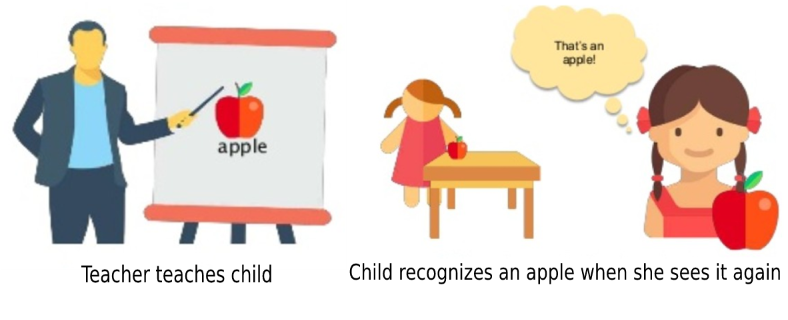The machine learning model is also able to learn from past data and make prediction/classification. This is called Supervised Learning.

Examples of Supervised Machine Learning:

Example 1:

• Task: Predicting the blood pressure of an adult based on his/her Age and Weight.
• Here Age and Weight are two predictors / input variables.
• Here Blood Pressure is the target / output variable.
• There are total 242 numbers of examples present in the dataset.
• Each training sample is of the form: [(Age, Weight), Blood Pressure]
• In the supervised learning framework, we shall use a large fraction of our data to “teach” the model, so that the model can learn and predict the rest of the data with high confidence.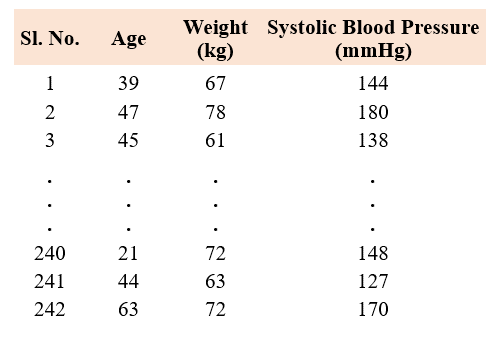Example 2:

• Task: Whether to sanction Loan or not to a person based on his/her Age, Monthly Income and Existing Loan amount.
• Here the predictor variables are Age, Monthly Income and Existing Loan and the target variable is Loan Sanction.
• There are total 1000 labelled samples given.
• Each sample is of the form: [(Age, Monthly Income, Existing Loan), Loan Sanction]
• Like previous, here also we take a fraction of the total dataset as training samples to train our supervised learning model.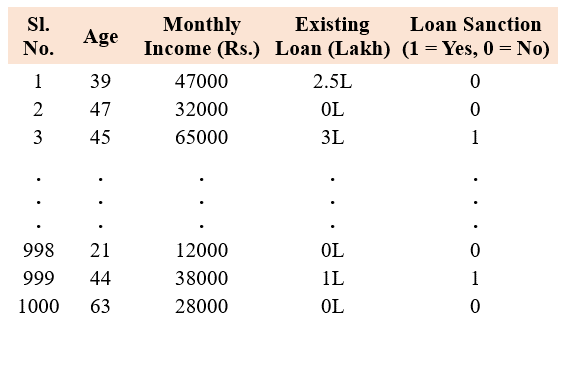What is the main difference between the examples of Supervised Learning we just saw?

• In the first example of “Blood pressure prediction” , the predicted or output variable assumes continuous values within a given range. This type of supervised learning is known as Prediction or Regression problem.
• While in the second example of “whether to sanction loan or not” , the predicted or output variable is categorical in nature, as it assumes only finite discrete values. This type of supervised learning is known as Classification problem.

Unsupervised Machine Learning:

Learning without teacher (self-organization)

Unsupervised learning is the machine learning task of inferring a function to describe inherent hidden structure from unlabelled data.Natural grouping of objects into two or more groups such that each object fall into exactly one group is called Clustering.

The word natural is not quite definitive. It can have different meanings. Hence different types of clusters possible out of same dataset.

Apart from clustering, there are other unsupervised learning techniques. One of such is Dimensionality Reduction. . In dimensionality reduction, we try to find out a lower order representation of the data without loosing much information.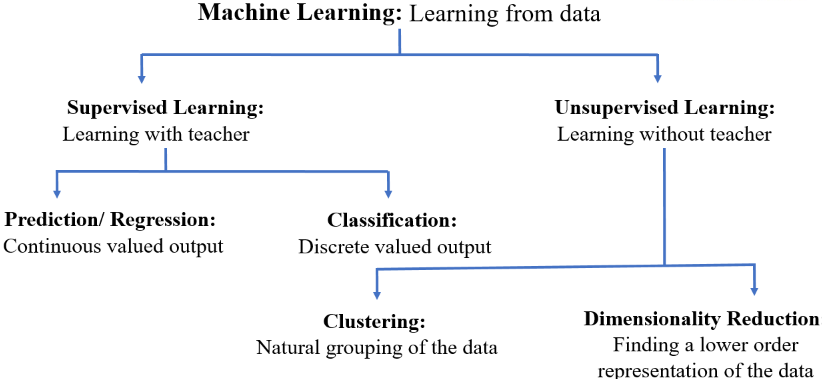Machine Learning Process:

Machine Learning is a data-oriented discipline. So, we need data to start a Machine Learning process. Usually more data leads to better algorithm.

• First identify the task and acquire the required data for the task.
• Then pre-process and clean the data.
• If it’s a supervised learning task then, given the pre-processed data first break it into train and test datasets: (Like 70% for training and 30% for test)
• Train your model on the training dataset and test it on the test dataset.
• Keep changing your model / model parameters until you get desired accuracy on both training and test sets.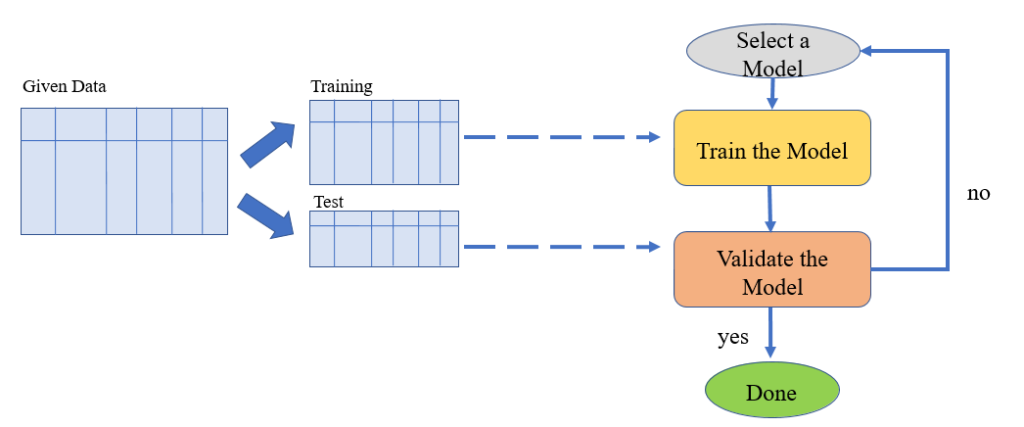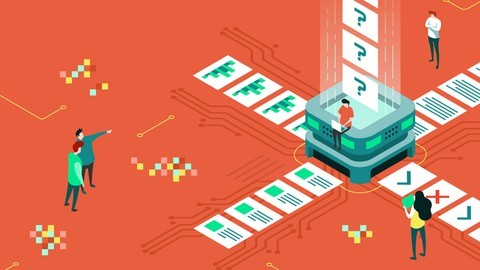Machine Learning Classification Bootcamp in Python